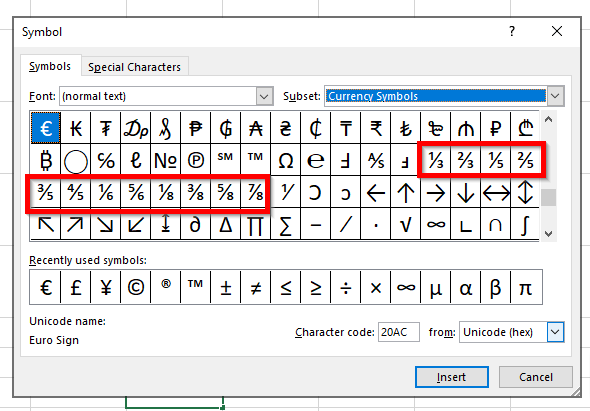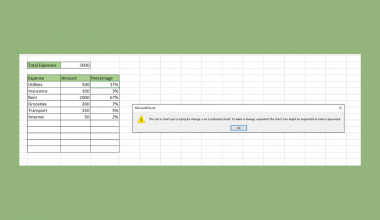# How to Add a Stacked Fraction in Excel

This tutorial will explain how you can add a stacked fraction in Excel.

Excel supports fractions as a number format, but this guide will explore other ways we can display a particular fraction value.

While decimals are the default format when working with numerical values in Excel spreadsheets, you may still encounter fractional values in a dataset. For example, when working with recipes, instructions may require a ½  teaspoon of salt or ¾  cup of rice.

Excel includes formatting options to display numerical values as fractions. However, users may find the need to represent fractions in a different format. For example, users may prefer to show a fraction in a stacked or vertical format, as seen below.Users may also opt for a skewed format for their fractions. These fractions are written on a downward angle with the diagonal fraction placed down and to the right of the numerator.To achieve these formats, users can use either the Equation editor or Symbol Menu to add stacked fractions and skewed fractions to their worksheets. The output of these options, however, is not treated as numerical values by Excel.

Now that we know what tools to use to add stacked and skewed fractions let’s see how they look on an actual sample spreadsheet.

## A Real Example of Using a Stacked Fraction in Excel

Let’s take a look at a sample spreadsheet that uses multiple methods to add stacked and skewed fractions.

The table below showcases multiple ways to add a stacked fraction in Excel. In the first column, we have the decimal value of the fraction, rounded off to the nearest hundredths.Column B is the only column of values that are formatted from numerical data. Since the data hold numerical values, users can perform operations on these fractions. You can learn how to get the values seen in column B by following our guide on how to convert decimals to fractions in Excel.

Columns C and D show two different equations that can be used to represent fractions. These equations are generated through Excel’s built-in Equation editor.

Column E consists of symbols or special characters that can be used to represent a select number of fractions.

If you’re ready to try out these methods in Excel, let’s begin writing it ourselves in the next section!

## How to Add a Stacked Fraction in Excel

This section will guide you through each step needed to add a stacked fraction in Excel. You’ll learn how you can use both the Equation editor and Symbol Menu to add stacked fractions and skewed fractions to your worksheet.

Follow these steps to start using the Equation editor in Excel:

1. First, navigate to the Insert tab and click on the Equation icon.2. You will now be able to see a new tab labeled ‘Equation’. In the Equation tab, click on the Fraction icon to see all possible formats you can use to generate your fraction.3. Once we select a format, an equation object will appear in your spreadsheet. You can drag and resize this object as needed.4. Next, specify the numerator and denominator of your fraction. In the example below, we’ve created the fraction ¾.5. Repeat the previous four steps to generate new fractions as needed. In the example below, we’ve compared two formats you can use with the Equation editor.While the Equation editor is a powerful tool for creating fractions, common fractions such as ½ and ⅔ have symbols that you can easily insert into your sheet with the Symbol menu.

Follow the instructions below to learn how to use the Symbol menu to add common stacked fractions:

1. In the Insert tab, click on the Symbol icon to open a new dialog box.2. In the Symbol dialog box, you can add characters representing stacked fractions.  Excel supports only a limited number of fractions as symbols.3. Click on the Insert button to add the character to the selected cell.4. Users can keep repeating the previous steps to add the necessary fraction values. An advantage of using symbols over equations is that we can easily use them as text values.1. Can I use equations or symbols with mathematical functions?
Equations are different from formulas. Equations do not perform calculations. To use fractions with actual operations, you can simply apply number formatting to existing decimal values.

This step-by-step guide should be all you need to begin adding stacked fractions and skewed fractions yourself in Excel. We’ve shown how easy it is to use equations and symbols to represent fractions in different formats in Excel.

The multiple methods we’ve explained show how flexible Excel can be in various situations. With so many other Excel functions available, you can surely find one that suits your use case.

Are you interested in learning more about what Microsoft Excel can do? Subscribe to our newsletter to find out about the latest Excel guides and tutorials from us.### Get emails from us about Excel.

Our goal this year is to create lots of rich, bite-sized tutorials for Excel users like you. If you liked this one, you'd love what we are working on! Readers receive ✨ early access ✨ to new content.

##### You May Also Like## How to Calculate Standard Error in Excel

This guide will discuss how to calculate the standard error in Excel using two easy methods. Excel is…## How to Select Non Adjacent Cells in Excel

This guide will show several methods that you can use to select non-adjacent cells in Excel. Non-adjacent cells…## How to Protect Formulas Without Protecting Worksheet in Excel

This guide will explain how you can protect formulas without protecting worksheets in Excel. You can protect cells…## How to Average Only Positive or Negative Numbers in Excel

You can use the AVERAGEIF function to average only positive or negative numbers in Excel. Computing the average…